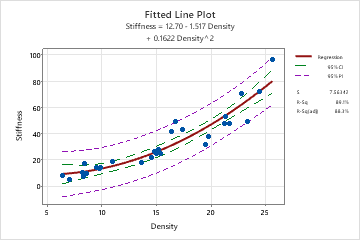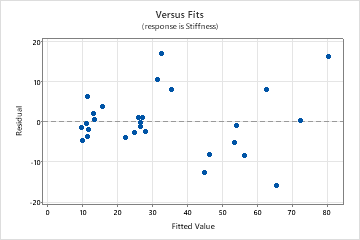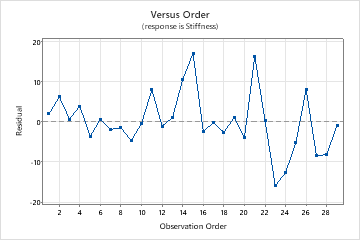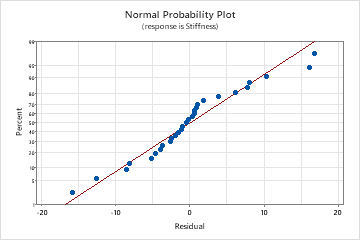# Interpret the key results for Fitted Line Plot

Complete the following steps to interpret a fitted line plot. Key output includes the p-value, the fitted line plot, R2, and the residual plots.

## Step 1: Determine whether the association between the response and the term is statistically significant

To determine whether the association between the response and each term in the model is statistically significant, compare the p-value for the term to your significance level to assess the null hypothesis. The null hypothesis is that the term's coefficient is equal to zero, which indicates that there is no association between the term and the response. Usually, a significance level (denoted as α or alpha) of 0.05 works well. A significance level of 0.05 indicates a 5% risk of concluding that an association exists when there is no actual association.
P-value ≤ α: The association is statistically significant
If the p-value is less than or equal to the significance level, you can conclude that there is a statistically significant association between the response variable and the term. If you fit a quadratic model or a cubic model and the quadratic or cubic terms are significant, you can conclude that the data contain curvature.
P-value > α: The association is not statistically significant

If the p-value is greater than the significance level, you cannot conclude that there is a statistically significant association between the response variable and the term. If you fit a quadratic model or a cubic model and the quadratic or cubic terms are not statistically significant, you may want to select a different model.

## Step 2: Determine whether the regression line fits your data

Evaluate how well the model fits your data and whether the model meets your goals. Examine the fitted line plot to determine whether the following criteria are met:
• The sample contains an adequate number of observations throughout the entire range of all the predictor values.
• The model properly fits any curvature in the data. If you fit a linear model and see curvature in the data, repeat the analysis and select the quadratic or cubic model. To determine which model is best, examine the plot and the goodness-of-fit statistics. Check the p-value for the terms in the model to make sure they are statistically significant, and apply process knowledge to evaluate practical significance.
• Look for any outliers, which can have a strong effect on the results. Try to identify the cause of any outliers. Correct any data entry or measurement errors. Consider removing data values that are associated with abnormal, one-time events (special causes). Then, repeat the analysis. For more information on detecting outliers, go to Unusual observations.
On this fitted line plot, the points generally follow the regression line. The points adequately cover the entire range of density values. However, the point in the top right corner of the graph appears to be an outlier. Investigate this point to determine its cause.## Step 3: Examine how the term is associated with the response

If the p-value of the term is significant, you can examine the regression equation and the coefficients to understand how the term is related to the response.

Use the regression equation to describe the relationship between the response and the terms in the model. The regression equation is an algebraic representation of the regression line. The regression equation for the linear model takes the following form: Y= b0 + b1x1. In the regression equation, Y is the response variable, b0 is the constant or intercept, b1 is the estimated coefficient for the linear term (also known as the slope of the line), and x1 is the value of the term.

The coefficient of the term represents the change in the mean response for one-unit change in that term. The sign of the coefficient indicates the direction of the relationship between the term and the response. If the coefficient is negative, as the term increases, the mean value of the response decreases. If the coefficient is positive, as the term increases, the mean value of the response increases.

For example, a manager determines that an employee's score on a job skills test can be predicted using the regression model, y = 130 + 4.3x. In the equation, x is the hours of in-house training (from 0 to 20) and y is the test score. The coefficient, or slope, is 4.3, which indicates that, for every hour of training, the mean test score increases by 4.3 points.

For more information on coefficients, go to Regression coefficients.

## Step 4: Determine how well the model fits your data

To determine how well the model fits your data, examine the goodness-of-fit statistics in the Model Summary table.

R-sq

R2 is the percentage of variation in the response that is explained by the model. The higher the R2 value, the better the model fits your data. R2 is always between 0% and 100%.

R2 always increases when you add additional predictors to a model. For example, the best five-predictor model will always have an R2 that is at least as high as the best four-predictor model. Therefore, R2 is most useful when you compare models of the same size.

Use adjusted R2 when you want to compare models that have different numbers of predictors. R2 always increases when you add a predictor to the model, even when there is no real improvement to the model. The adjusted R2 value incorporates the number of predictors in the model to help you choose the correct model.

Consider the following when you compare the R2 values:
• Small samples do not provide a precise estimate of the strength of the relationship between the response and predictors. For example, if you need R2 to be more precise, you should use a larger sample (typically, 40 or more).

• Goodness-of-fit statistics are just one measure of how well the model fits the data. Even when a model has a desirable value, you should check the residual plots to verify that the model meets the model assumptions.

## Step 5: Determine whether your model meets the assumptions of the analysis

Use the residual plots to help you determine whether the model is adequate and meets the assumptions of the analysis. If the assumptions are not met, the model may not fit the data well and you should use caution when you interpret the results.

### Residuals versus fits plot

Use the residuals versus fits plot to verify the assumption that the residuals are randomly distributed and have constant variance. Ideally, the points should fall randomly on both sides of 0, with no recognizable patterns in the points.

The patterns in the following table may indicate that the model does not meet the model assumptions.
Pattern What the pattern may indicate
Fanning or uneven spreading of residuals across fitted values Nonconstant variance
Curvilinear A missing higher-order term
A point that is far away from zero An outlier
A point that is far away from the other points in the x-direction An influential point
In this residuals versus fits plot, the points appear randomly scattered on the plot. However, the point in the upper right corner appears to be an outlier. Try to identify the cause of the outlier. Correct any data entry or measurement errors. Consider removing data values that are associated with abnormal, one-time events (special causes). Then, repeat the analysis.### Residuals versus order plot

Use the residuals versus order plot to verify the assumption that the residuals are independent from one another. Independent residuals show no trends or patterns when displayed in time order. Patterns in the points may indicate that residuals near each other may be correlated, and thus, not independent. Ideally, the residuals on the plot should fall randomly around the center line:If you see a pattern, investigate the cause. The following types of patterns may indicate that the residuals are dependent.
In this residuals versus order plot the points appear to fall randomly around the centerline. Points that correspond to the observations in rows 15, 21, and 23 of the worksheet have larger residuals.### Normal probability plot

Use the normal probability plot of the residuals to verify the assumption that the residuals are normally distributed. The normal probability plot of the residuals should approximately follow a straight line.

The patterns in the following table may indicate that the model does not meet the model assumptions.
Pattern What the pattern may indicate
Not a straight line Nonnormality
A point that is far away from the line An outlier
Changing slope An unidentified variable
In this normal probability plot, the residuals deviate slightly from the straight line. However, when the data contain at least 15 points, normality is usually not a problem for a reliable p-value calculation.For more information on how to handle patterns in the residual plots, go to Residual plots for Fitted Line Plot.

By using this site you agree to the use of cookies for analytics and personalized content.  Read our policy